Miscellaneous

Chapter 8 Class 11 Binomial Theorem (Deleted)
Serial order wise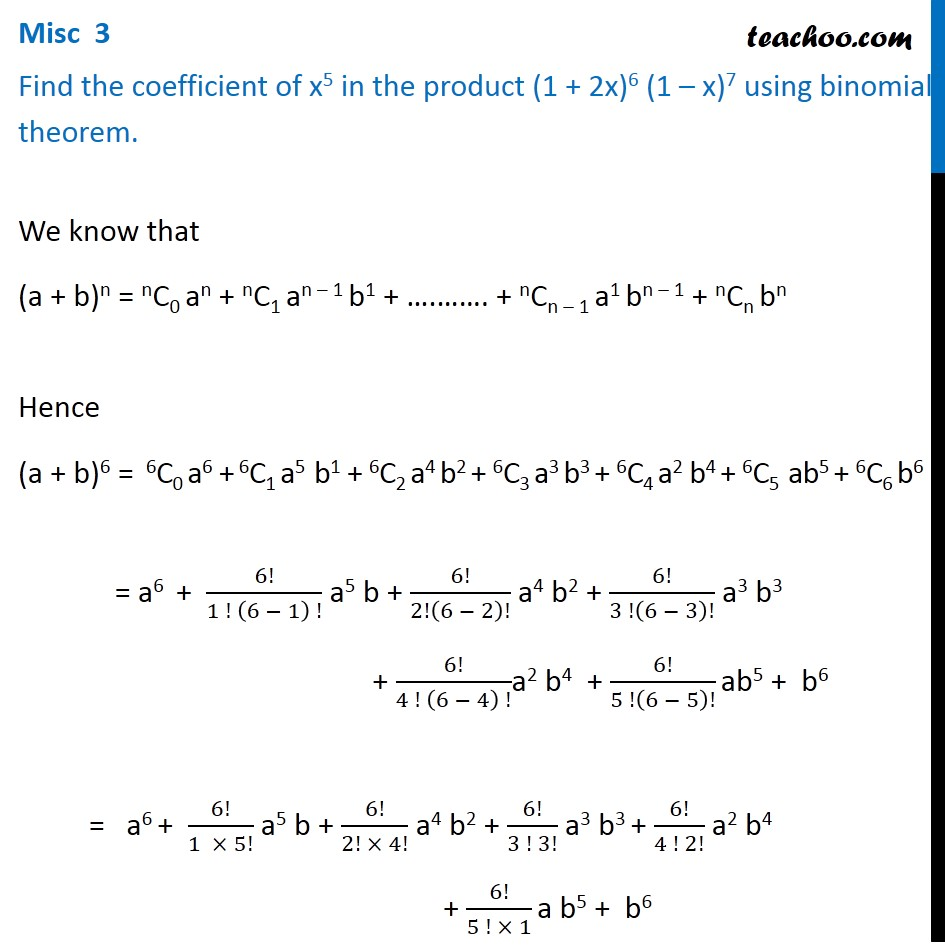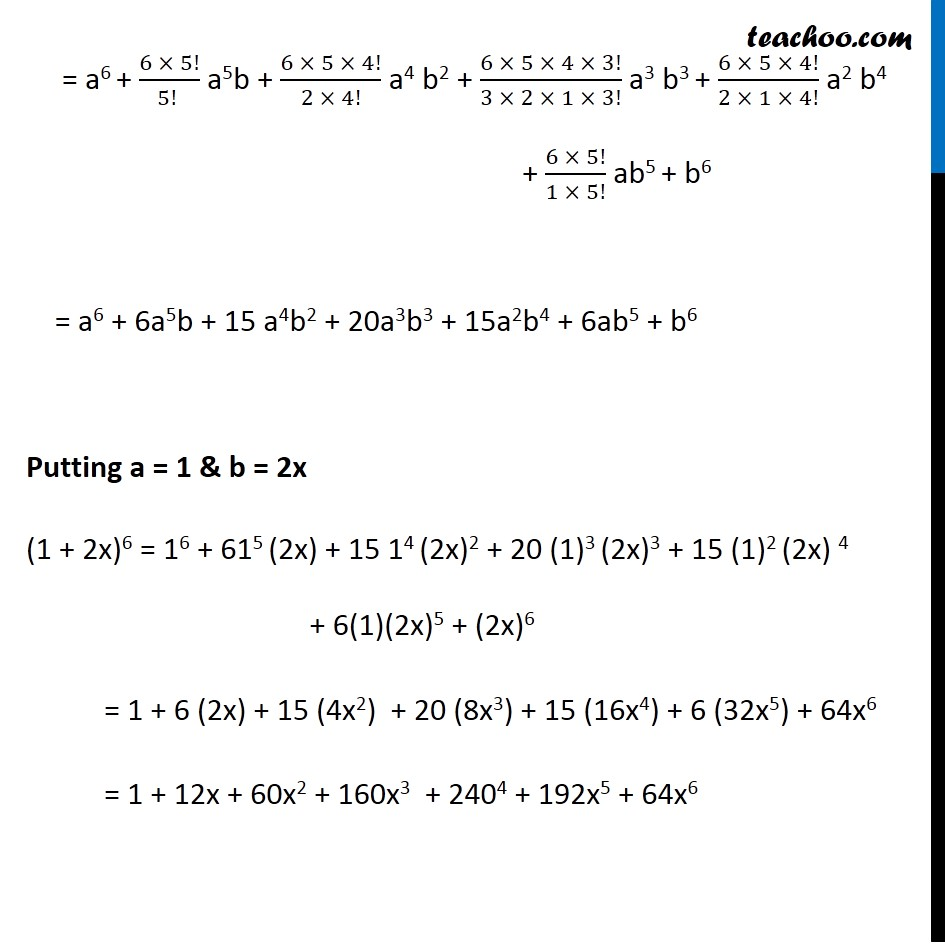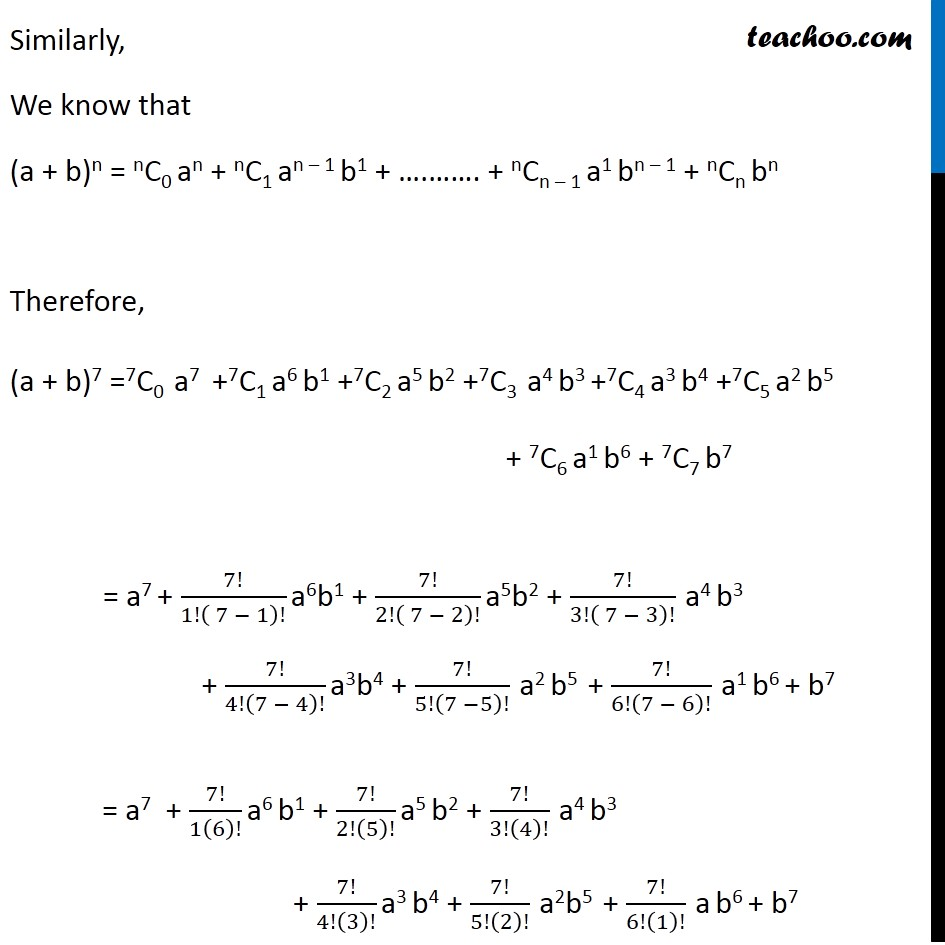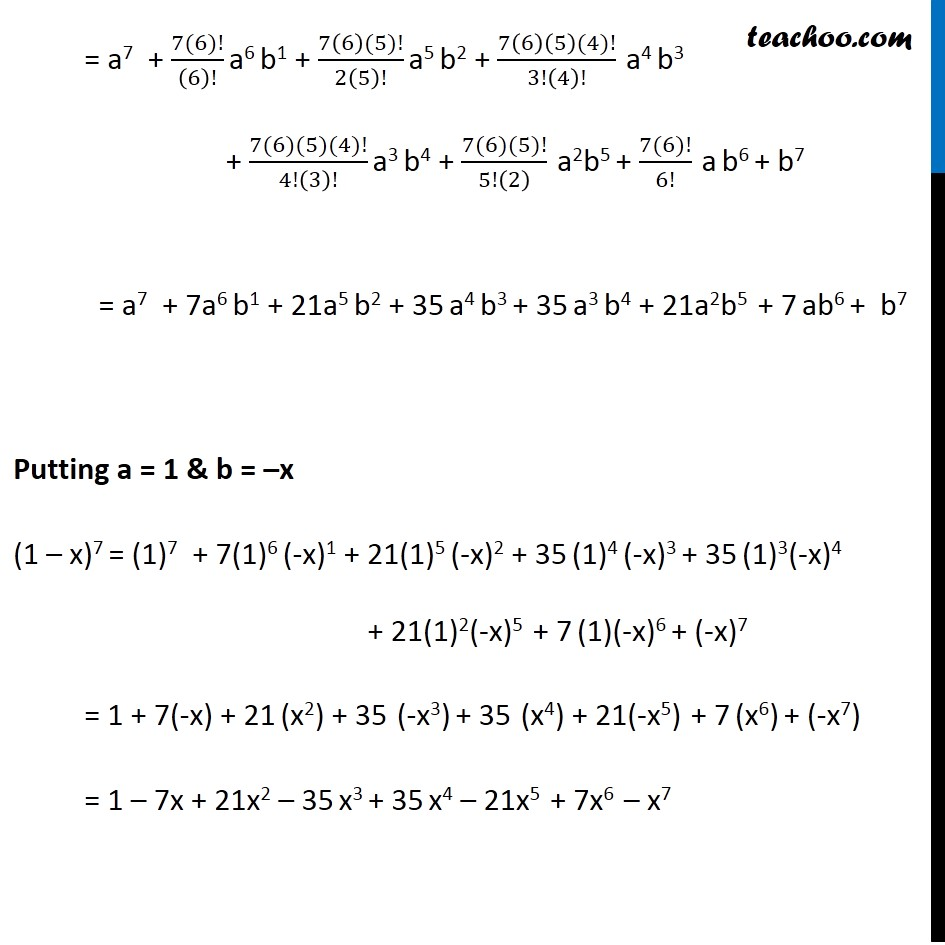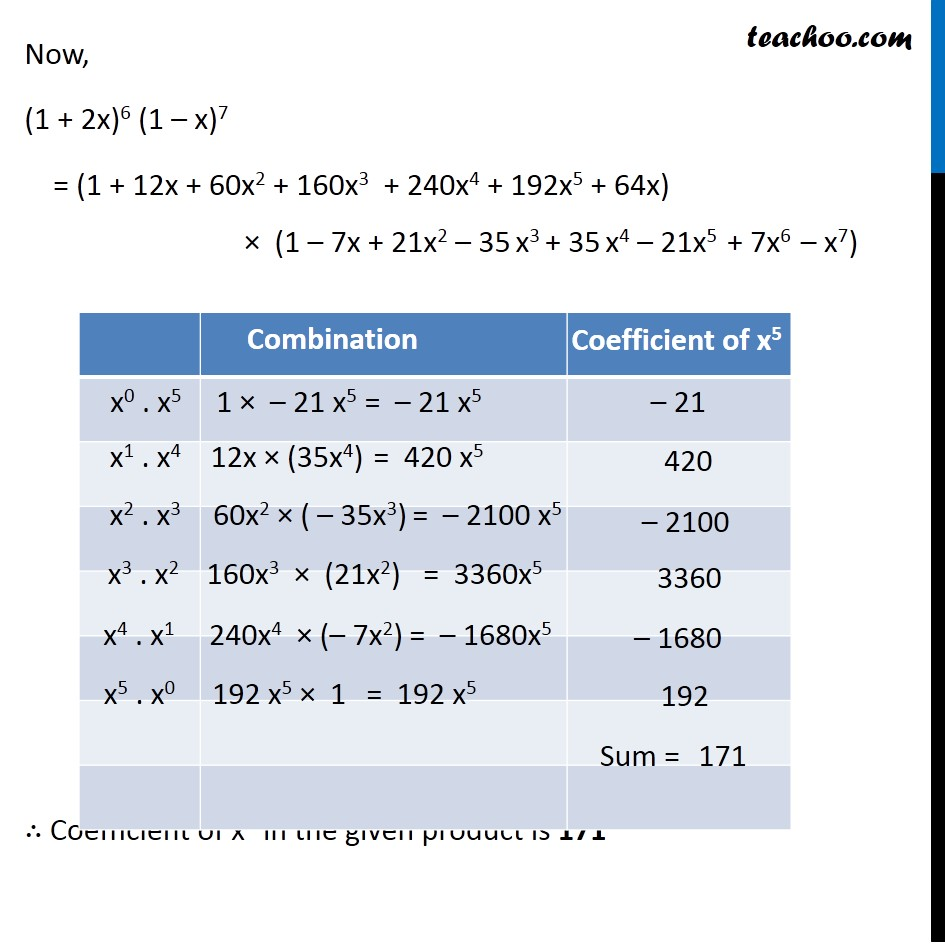549 people joined Teachoo Black. What are you waiting for?

### Transcript

Misc 3 Find the coefficient of x5 in the product (1 + 2x)6 (1 – x)7 using binomial theorem. We know that (a + b)n = nC0 an + nC1 an – 1 b1 + ….……. + nCn – 1 a1 bn – 1 + nCn bn Hence (a + b)6 = 6C0 a6 + 6C1 a5 b1 + 6C2 a4 b2 + 6C3 a3 b3 + 6C4 a2 b4 + 6C5 ab5 + 6C6 b6 = a6 + 6!/(1 ! (6 − 1) !) a5 b + 6!/2!(6 − 2)! a4 b2 + 6!/(3 !(6 − 3)!) a3 b3 + 6!/(4 ! (6 − 4) !)a2 b4 + 6!/(5 !(6 − 5)!) ab5 + b6 = a6 + 6!/(1 × 5!) a5 b + 6!/(2! × 4!) a4 b2 + 6!/(3 ! 3!) a3 b3 + 6!/(4 ! 2!) a2 b4 + 6!/(5 ! × 1) a b5 + b6 = a6 + (6 × 5!)/(5! ) a5b + (6 × 5 × 4!)/(2 × 4!) a4 b2 + (6 × 5 × 4 × 3!)/(3 × 2 × 1 × 3!) a3 b3 + (6 × 5 × 4!)/(2 × 1 × 4!) a2 b4 + (6 × 5!)/(1 × 5!) ab5 + b6 = a6 + 6a5b + 15 a4b2 + 20a3b3 + 15a2b4 + 6ab5 + b6 Putting a = 1 & b = 2x (1 + 2x)6 = 16 + 615 (2x) + 15 14 (2x)2 + 20 (1)3 (2x)3 + 15 (1)2 (2x) 4 + 6(1)(2x)5 + (2x)6 = 1 + 6 (2x) + 15 (4x2) + 20 (8x3) + 15 (16x4) + 6 (32x5) + 64x6 = 1 + 12x + 60x2 + 160x3 + 2404 + 192x5 + 64x6 Similarly, We know that (a + b)n = nC0 an + nC1 an – 1 b1 + ….……. + nCn – 1 a1 bn – 1 + nCn bn Therefore, (a + b)7 =7C0 a7 +7C1 a6 b1 +7C2 a5 b2 +7C3 a4 b3 +7C4 a3 b4 +7C5 a2 b5 + 7C6 a1 b6 + 7C7 b7 = a7 + 7!/1!( 7 − 1)! a6b1 + 7!/2!( 7 − 2)! a5b2 + 7!/3!( 7 − 3)! a4 b3 + 7!/4!(7 − 4)! a3b4 + 7!/5!(7 −5)! a2 b5 + 7!/6!(7 − 6)! a1 b6 + b7 = a7 + 7!/1(6)! a6 b1 + 7!/2!(5)! a5 b2 + 7!/3!(4)! a4 b3 + 7!/4!(3)! a3 b4 + 7!/5!(2)! a2b5 + 7!/6!(1)! a b6 + b7 = a7 + (7(6)!)/(6)! a6 b1 + (7(6)(5)!)/2(5)! a5 b2 + (7(6)(5)(4)!)/3!(4)! a4 b3 + (7(6)(5)(4)!)/4!(3)! a3 b4 + (7(6)(5)!)/5!(2) a2b5 + (7(6)!)/6! a b6 + b7 = a7 + 7a6 b1 + 21a5 b2 + 35 a4 b3 + 35 a3 b4 + 21a2b5 + 7 ab6 + b7 Putting a = 1 & b = –x (1 – x)7 = (1)7 + 7(1)6 (-x)1 + 21(1)5 (-x)2 + 35 (1)4 (-x)3 + 35 (1)3(-x)4 + 21(1)2(-x)5 + 7 (1)(-x)6 + (-x)7 = 1 + 7(-x) + 21 (x2) + 35 (-x3) + 35 (x4) + 21(-x5) + 7 (x6) + (-x7) = 1 – 7x + 21x2 – 35 x3 + 35 x4 – 21x5 + 7x6 – x7 Now, (1 + 2x)6 (1 – x)7 = (1 + 12x + 60x2 + 160x3 + 240x4 + 192x5 + 64x) × (1 – 7x + 21x2 – 35 x3 + 35 x4 – 21x5 + 7x6 – x7) ∴ Coefficient of x5 in the given product is 171